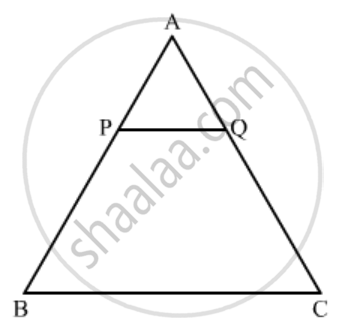Advertisement Remove all ads

# In the Given Figure, Pq || Bc and Ap : Pb = 1 : 2. Find a R E a ( δ a P Q ) a R E a ( δ a B C ) - Mathematics

Sum

In the given figure, PQ || BC and AP : PB = 1 : 2. Find$\frac{area \left( ∆ APQ \right)}{area \left( ∆ ABC \right)}$Advertisement Remove all ads

#### Solution

GIVEN: In the given figure PQ || BC, and AP: PB = 1:2

TO FIND:$\frac{area \left( ∆ APQ \right)}{area \left( ∆ ABC \right)}$

We know that according to basic proportionality theorem if a line is drawn parallel to one side of a triangle intersecting the other side, then it divides the two sides in the same ratio.

Since triangle APQ and ABC are similar

Hence (AP)/(AB)=(AQ)/(AC)=(PQ)/(BC)

Now, it is given that (AP)/(PB)=1/2.

⇒ PB =2AP

(AP)/(AB)=(AP)/(AP+PB)=(AP)/(AP+2AP)=1/3

Since the ratio of the areas of two similar triangle is equal to the ratio of the squares of their corresponding sides.

$\frac{Area\left( APQ \right)}{Area\left( ABC \right)} = \left( \frac{AP}{AB} \right)^2 = \left( \frac{1}{3} \right)^2 = \frac{1}{9}$

Hence we got the result Area (Δ APB):Area(ΔABC)=1:9

Concept: Triangles Examples and Solutions
Is there an error in this question or solution?
Advertisement Remove all ads

#### APPEARS IN

RD Sharma Class 10 Maths
Chapter 7 Triangles
Q 17 | Page 130
Advertisement Remove all ads

#### Video TutorialsVIEW ALL 

Advertisement Remove all ads
Share
Notifications

View all notifications

Forgot password?
Course